# Approximating Integrals

 Today: 7.7 - approximating integrals Friday: Third QUIZ and 7.8 - improper integrals

Problem: Compute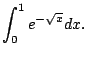Hmmm... Any ideas?

Today we will revisit Riemann sums in the context of finding numerical approximations to integrals, which we might not be able to compute exactly. Recall that if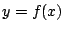then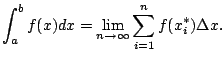The fundamental theorem of calculus says that if we can find an antiderivative of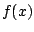, then we can compute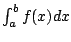exactly. But antiderivatives can be either (1) hard to find, and sometimes worse (2) impossible to find. However, we can always approximate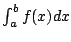(possibly very badly).

For example, we could use Riemann sums to approximate, say using left endpoints. This gives the approximation: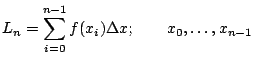left endpoints

Using rightpoints gives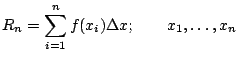right endpoints

Using midpoints gives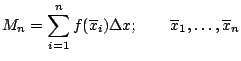midpointswhere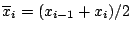. The midpoint is typically (but not always) much better than the left or right endpoint approximations.

Yet another possibility is the trapezoid approximation, which is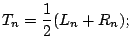this is just the average of the left and right approximations.

Question 5.6.1   But wouldn't the trapezoid and midpoint approximations be the same?-certainly not (see example below); interestingly, very often the midpoint approximation is better.

Simpson's approximation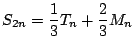gives the area under best-fit parabolas that approximate our function on each interval. The proof of this would be interesting but takes too much time for this course.

Many functions have no elementary antiderivatives: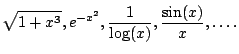NOTE - they do have antiderivatives; the problem is just that there is no simple formula for them. Why are there no elementary antiderivatives?

Some of these functions are extremly important. For example, the integrals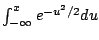are extremely important in probability, even though there is no simple formula for the antiderivative.

If you are doing scientific research you might spend months tediously computing values of some function, for which no formula is known.

Example 5.6.2   Compute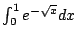.
1. Trapezoid with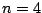2. Midpoint with3. Simpson's with with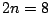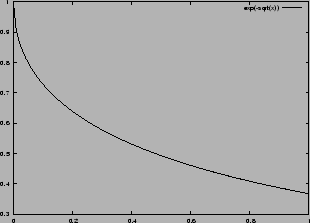The following is a table of the values ofat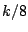for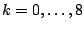.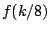0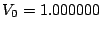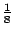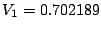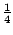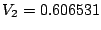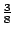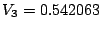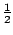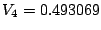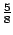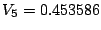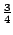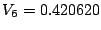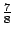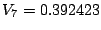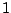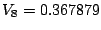<>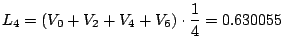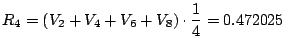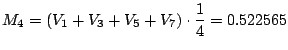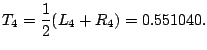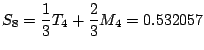Maxima gives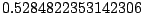and Mathematica gives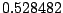.

Note that Simpsons's is the best; it better be, since we worked the hardest to get it!

 Method Error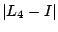0.101573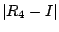0.056458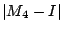0.005917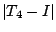0.022558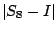0.003575
<>

William Stein 2006-03-15